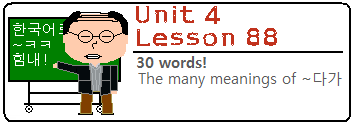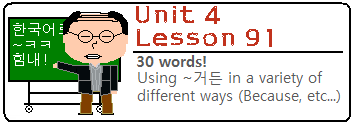Lesson 84: In this lesson, you will learn about the common grammatical principle ~자마자 and its less common counterpart, ~자. Both have similar meanings, but as always I will dissect each one so you can understand them more clearly by the end of this lesson. This Lesson is also available in EspañolLesson 85: In this lesson, you will learn two grammatical principles that can be used with the verb 알다 (to know). First, you will learn about ~ㄹ/을 줄 알다 [/모르다] (to know how) and second you will learn about 알다시피 (as you know). This Lesson is also available in EspañolLesson 86: In this lesson, you will learn how to use the word 아니라 (from the word 아니다) to negate a preceding noun. In addition, you will also learn how this same grammatical principle can negate an entire clause ending in an adjective or verb in the form of ~는 게 아니라. This Lesson is also available in EspañolLesson 87: In this lesson, you will learn how to add ~기로 하다 to the end of a sentence or clause to have the meaning “to decide to.” The usage and translation are fairly straightforward, to the point that the construction can almost be thought of as an idiom. However, I do feel that this is a grammatical principle that deserves its own lesson. This Lesson is also available in EspañolLesson 88: In this lesson, you will learn about the various usages of ~다가, which is often placed between two clauses. The difficulty with ~다가 is that there are many meanings that this grammatical principle can take on – all with a seemingly identical purposes. Because of this confusion, this grammatical principle has plagued learners of Korean for years. Let’s get started. This Lesson is also available in EspañolLesson 89: In this lesson, you will learn a variety of new words and grammatical principles that you can use in comparative sentences. You will learn about 비교하다 and ~에 비해 and how to use fractions and how to say things like “two times better” or “half as good,” which are commonly used in comparison sentences. This Lesson is also available in EspañolLesson 90: In this lesson you will learn how to end sentences with ~잖다 (~잖아 or ~잖아요). Though this is a very common ending in conversational Korean, English speakers often cannot figure out exactly what it means. The main reason for this is simply because it is hard to translate directly into English. In this lesson, there will be many examples that describe specifically the situation where this grammatical form can be used. This Lesson is also available in EspañolLesson 91: In this lesson, you will learn how to add ~거든(요) to the end of a sentence or to connect two clauses. Though this grammatical form has one very common meaning, it is often used to have other meanings as well. In this lesson, I will break down all the meanings of ~거든(요) and explain how they can be used. This Lesson is also available in EspañolLessons 84 – 91 Mini Test: Test yourself on what you learned from Lessons 84 to 91! This Mini-Test is also available in Español